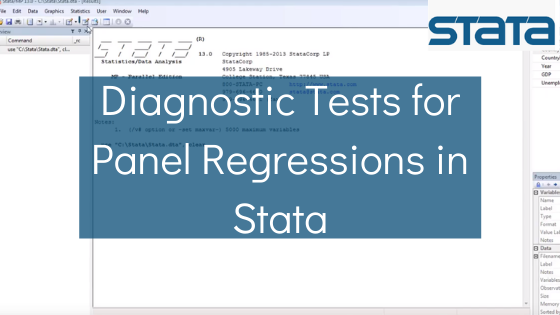Phone: (+44) 0203 9500 830

Whatsapp: (+44) 0794 0000 629

# Diagnostic Tests for Panel Regressions in Stata## Overview

When performing a panel regression analysis in Stata, additional diagnostic tests are run to detect potential problems with residuals and model specification. In order to rely on the estimated coefficients and consider them accurate representations of true parameters, it is important that the assumptions of linear regressions formulated in the Gauss-Markov theorem should be met. Most of the assumptions relate to the characteristics of the regression residuals. The latter should be independent, without serial correlation and homoscedastic. The latter means the residuals should have equal variance.

## Diagnostics Tests

Unlike traditional OLS regressions, panel regression analysis in Stata does not come with a good choice of diagnostic tests such as the Breusch-Pagan test for panel regressions. Additional user written modules have to be downloaded to conduct heteroscedasticity tests (e.g. httest2, httest3, etc.). Some researchers prefer to ignore these tests. Heteroscedasticity is a problem often found in cross-sectional regressions but in panel regressions both cross-sections and time-series are used. Other researchers download use-written modules and test for this problem. Yet others estimate panel regressions with clustered standard errors. This option is available in Stata. This would help researchers to eliminate heteroscedasticity if it exists.

## Serial Correlation

Similar to the heteroscedasticity test, serial correlation in panel regressions is also tested by downloading user-written modules in Stata. The default functions of Stata do not provide such options for diagnostic testing. A well-known user-written programme that can be run in Stata to detect serial correlation in panel regressions is xtserial. Serial correlation is often found in time-series and rarely observed in cross-sectional data. In panel regressions, serial correlation could be caused by seasonal effects and non-stationarity of the data inputs. Stationarity can be tested by running panel data unit root tests in Stata. If potential endogeneity issues are detected in the relationship between dependent and independent variable, an instrumental variable (IV) regression should be run instead of standard panel regressions.

## Final Note

Finally, the Hausman specification test for choosing the appropriate type of effects, either random effects (RE) or fixed effects (FE) should be conducted in Stata. The output of the FE specification is recorded first. Next, the output of the RE model is recorded. After that, the Hausman test is conducted. The null hypothesis of the test is that RE model produces consistent coefficients and therefore it should be preferred since it is more efficient than the FE specification. If the p-value of the test is higher than the significance level, this hypothesis is accepted. In the opposite case, the FE model is chosen instead. The latter is less efficient but always consistent.